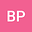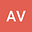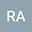An iterative method to compute dual solutions on a class of nonlinear higher order SBVPs arising in MBE
•••Biswajit Pandit
IIT Patna

Corresponding Author:[email protected]

Author ProfileRavi P. Agarwal
Texas A&M University -Kingsville
Author Profile## Abstract

In this work, we focus on the following non-linear fourth order SBVP \begin{eqnarray} \nonumber \frac{1}{r}\left[ r \left\lbrace \frac{1}{r} \left(r \phi’ \right)^{’} \right\rbrace^{’}\right]^{’}=\frac{\phi’ \phi’‘}{r}+\lambda, \end{eqnarray} where $\lambda$ is a parameter. We convert this non-linear differential equation into third order non-linear differential equation, which is given by \begin{eqnarray} \nonumber \frac{1}{r}\left[ r \left\lbrace \frac{1}{r} \left(r y \right)^{’} \right\rbrace^{’}\right]^{’}=\frac{y y’}{r}+\lambda. \end{eqnarray} The problem is singular, non self adjoint, nonlinear. Moreover, depending upon $\lambda$, it admits multiple solutions. Hence, it is too difficult to capture these solutions by any discrete method such as finite difference etc. Here we propose an iterative technique by using homotopy perturbation method (HPM) with the help of variational iteration method (VIM) in a suitable way. We compute these solutions numerically. Convergence of this series solution is studied in a novel way. For small positive values of $\lambda$, singular BVP has two solutions while solutions can not be found for large positive values of $\lambda$. Furthermore, we also find dual solutions for $\lambda <0$.